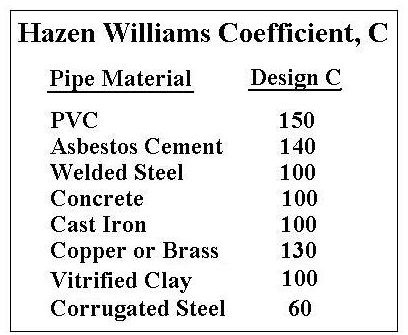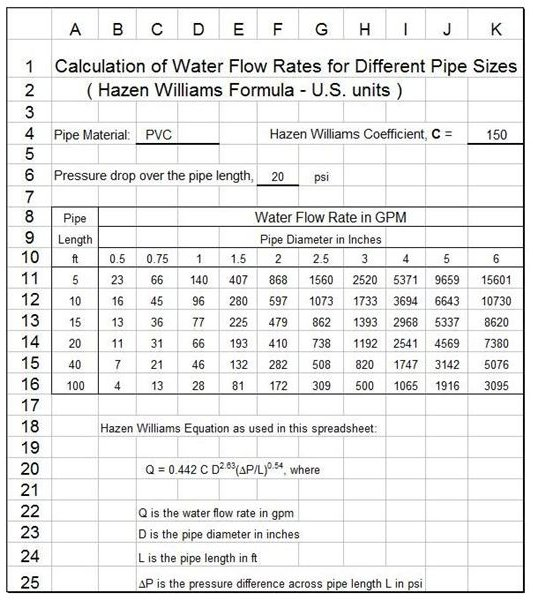# Water Flow Rates for Pipe Sizes with Excel Formulas, Using the Hazen Williams Formula

## Limitations on the Hazen Williams Formula for Water Flow Rate Calculations

The Hazen Williams formula is an empirical equation that can be used for turbulent flow of water at typical ambient temperatures. The turbulent flow requirement is not very limiting. Most practical applications of water transport in pipes are in the turbulent flow regime. For a review of this topic see the article, 'Reynolds Number and Laminar & Turbulent Flow.' Strictly speaking, the Hazen Williams formula applies to water at 60oF, but it works quite well for a reasonable range of water temperatures above or below 60oF. For fluids with viscosity different from water, or for water temperatures far above or below 60oF, the Darcy Weisbach Equation works better than the Hazen Williams Formula. Click on the following link for more details about the Darcy Weisbach Equation.

Following presentation and discussion of several forms of the Hazen Williams equation in the next couple of sections, a downloadable Excel spreadsheet template will be presented and discussed for making Hazen Williams water flow rate calculations, using Excel formulas.

## Forms of the Hazen Williams Formula

There are several different forms of the Hazen Williams Formula in use for water flow rate calculations. It can be written in terms of water velocity or water flow rate, in terms of pressure drop or head loss, and for several different sets of units. The traditional form of the Hazen Williams formula is:

U.S. units: V = 1.318 C R0.633 S0.54, where:

• V = water flow velocity in ft/sec
• C = Hazen Williams coefficient, dependent on the pipe material and pipe age
• R = Hydraulic radius, ft (R = cross-sectional area/wetted perimeter)
• S = slope of energy grade line = head loss/pipe length = hL/L, which is dimensionless

S.I. units: V = 0.85 C R0.633 S0.54, where:

• V is in m/s and R is in meters

The Hazen Williams Formula is used primarily for pressure flow in pipes, for which the hydraulic radius is one fourth of the pipe diameter (R = D/4). Using this relationship and Q = V(πD2/4), for flow in a circular pipe, the Hazen Williams formula can be rewritten as shown in the next section.

## Water Flow Rates for Pipe Sizes over a Range of Diameters with the Hazen Williams Formula

For flow of water under pressure in a circular pipe, the Hazen Williams formula shown above can be rewritten into the following convenient form:

in U.S. units: Q = 193.7 C D2.63 S0.54, where:

• Q = water flow rate in gal/min (gpm)
• D = pipe diameter in ft
• C and S are the same as above

in S.I. units: Q = 0.278 C D2.63 S0.54, where

• Q is in m3/s and D is in meters

The Hazen Williams formula can also be expressed in terms of the pressure difference (ΔP) instead of head loss (hL) across the pipe length, L, using ΔP = ρghL:

In S.I. units, a convenient form of the equation is: Q = (3.763 x 10-6) C D2.63 (ΔP/L)0.54, where

• Q is water flow rate in m3/hr,
• D is pipe diameter in mm
• L is pipe length in m,
• ΔP is the pressure difference across pipe length, L, in kN/m2

In U.S. units: Q = 0.442 C D2.63 (ΔP/L)0.54, where

• Q is water flow rate in gpm,
• D is pipe diameter in inches
• L is pipe length in ft,
• ΔP is the pressure difference across pipe length, L, in psi

This is a form of the Hazen Williams formula that is convenient to use for estimating water flow rates for pipe sizes and lengths in U.S. units, as illustrated in the section after next on the second page.

The second page of this article has a table with values for the Hazen Williams coefficient, a table with example water flow rate calculations for several PVC pipe lengths and diameters, and a link to download a spreadsheet template with Excel formulas to make the water flow rate calculations.

## Values for the Hazen Williams CoefficientIn order to use the Hazen Williams formula for water flow rate calculations, values of the Hazen Williams coefficient, C, are needed for the pipe material being used. Values of C are available in many handbooks, textbooks, and on internet sites. C values typically used for some common pipe materials are shown in the table at the left.

Source:Toro Ag Irrigation (PDF)

## Example Calculation of Water Flow Rates for Pipe Sizes and Lengths

The table below was prepared using the equation: Q = 0.442 C D2.63 (ΔP/L)0.54, with units as given above, to calculate the water flow rates for PVC pipe with diameters from 1/2 inch to 6 inches and length from 5 ft to 100 ft, all for a pressure difference of 20 psi across the particular length of pipe. The Hazen Williams coefficient was taken to be 150 per the table in the previous section.

………………………………………..WATER FLOW RATE IN GPM………………………………………

…………………. …………………………….Pipe Diameter in Inches………………………………………

length, ft……..0.5…….0.75……..1………1.5………2………2.5………3……….4……….5…………6

….5…………….23……..66……..140…….407…….868…..1560……2520….5371…..9659……15,601

10……………16……..45………96……..280…….597…..1073……1733….3694…..6643……10,730

15……………13……..36………77……..225…….479……862…….1393….2968…..5337……..8620

20……………11……..31………66……..193…….410……738…….1192….2541…..4569……..7380

40…………….7………21………46……..132…….282……508……..820…..1747…..3142……..5076

..100……………4………13………28………81……..172……309……..500…..1065…..1916……..3096

The table shows a pattern that you should intuitively expect. For a given pressure difference driving the flow, the water flow rate increases as diameter increases for a given pipe length and the water flow rate decreases as pipe length increases for a given pipe diameter. The equation above can be used to calculate water flow rates for pipe sizes and lengths with different pipe materials and pressure driving forces, using the Hazen Williams equation as demonstrated in the table above.

## An Excel Template to Calculate Water Flow Rates for Pipe Sizes and lengths.The spreadsheet template at the left has the Excel formulas built in to calculate water flow rates for different pipe sizes as illustrated in the previous section. This Excel spreadsheet template that can be downloaded below, allows for input of the Hazen Williams coefficient value and the pressure drop across the length of pipe being considered. Also, the pipe diameters and lengths can be changed from those currently in the spreadsheet, so the flow rate can be calculated for any combination of pipe diameter and length if the Hazen Williams coefficient is known and the pressure drop across the pipe is known.

The example spreadsheet has U.S. units, but an S.I. version and a U.S. version are available for download.

## References

References for Further Information:

1. Bengtson, H., Fundamentals of Fluid Flow, An online continuing education course for PDH credit.

2. Munson, B. R., Young, D. F., & Okiishi, T. H., Fundamentals of Fluid Mechanics, 4th Ed., New York: John Wiley and Sons, Inc, 2002.

3. Liou, C.P., "Limitations and Proper Use of the Hazen-Williams Equation," Journal of Hydraulic Engineering, Vol. 124, No. 9, Sept. 1998, pp. 951-954.

## This post is part of the series: Pipe Flow Calculations

Pipe flow calculations include using Reynolds number to find if the flow is laminar flow or turbulent flow. Frictional head loss can be found using the Darcy Weisbach equation and the friction factor. The entrance length for fully developed flow can be found for turbulent flow and for laminar flow.